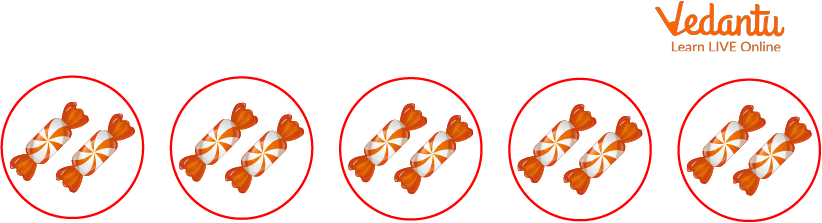Courses
Courses for Kids
Free study material
Free LIVE classes
More## Introduction: Activities Related to Repeated Addition

Repeated addition is a mathematical process of adding groups with equal numbers together. So, where do you use repeated addition in daily life? Repeated addition is very useful when counting notes with similar denominations. For example, you have to count 4, 20 Rs. coin. So, in that case, repeated addition helps a lot. It also helps in understanding multiplication better. So, let's learn repeated addition.

## What is the Meaning of Repeated Addition?

As mentioned above, repeated addition means adding a group containing an equal number of items or quantities. For example, have a look at the below image. There are 5 groups, with each group having 2 candies.5 Groups

So, how many candies are there in total?

To calculate these, we can write that,

2 + 2 + 2 + 2 + 2 = 10

However, repeated counting can be visualised with the help of the number line and skip counting.

Therefore, when you skip count by 2 using the number line 5 times, the final number you get is again 10.

## Relation of Repeated Addition and Multiplication

Repeated addition is related to multiplication. Let us take the previous example of repeated addition. Since there were 2 candies in each group. The total number of candies is 10. Now, since every group had an equal number of candies, that is 2. We can write that

$2 \times 5 = 10$

Since there were 5 groups, 2 was multiplied by 5. This is how multiplication is related to repeated addition.

## Classroom Activities for Teaching Repeated Addition

Here are some activities related to repeated addition for kids that can be used to make the kids understand the concept better.

1. Take 4 pencil boxes with 3 pencils in each box and then ask the kids to determine the total number of pencils for 4 boxes.

2. Give the children 5 packets, with each packet containing 4 candies. Ask the children to count the number of candies in all the packets after they are done counting. Ask them to use the multiplication trick for repeated addition and verify whether the answer in both cases is the same.

3. Give the children 3 matchboxes with 5 matchsticks in each box. Ask them to count and find the total number of match sticks in all the boxes. After that, ask them to perform repeated addition and find the answer.

With the help of the above activities, you can easily make the kids understand what repeated addition is.

## Conclusion

So, today you have learnt about repeated addition. You have learnt that repeated addition is adding groups with the same numbers or quantities more than once. You have also learned the relationship between repeated addition, skip counting, and multiplication. Apart from these concepts, you found some classroom activities that can be performed in classes to understand repeated addition better.

Last updated date: 20th Sep 2023
Total views: 76.8k
Views today: 1.76k

## FAQs on Activities for Repeated Addition

1. What is repeated addition commonly known as?

Repeated addition is also known as multiplication. Repeated addition activities are just a way for children to learn and understand the real meaning of multiplication. Repeated addition activities also help a lot in understanding multiplication.

2. Is repeated addition similar to skip counting?

Repeated addition is similar to skip counting. However, skip counting is a number line where a particular number is skipped many times to find the final number. In repeated addition, the particular number is added repeatedly to find the final number. Both methods use different techniques but are similar in giving the same answer.

3. Are repeated addition activities necessary for a child?

Repeated addition activities are necessary for a child, as they help the child understand advanced concepts like multiplication. Simple activities related to repeated addition are easy to understand and help with better understanding.